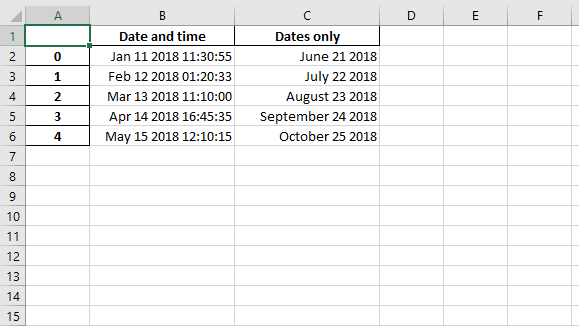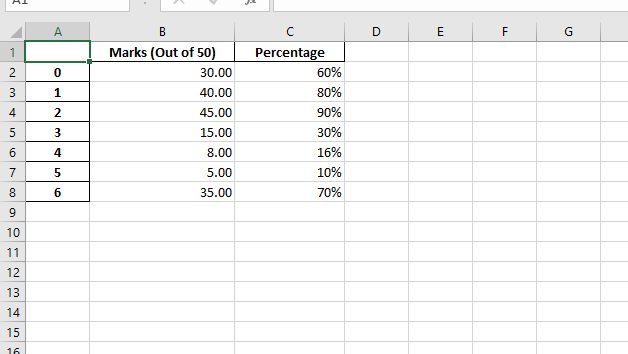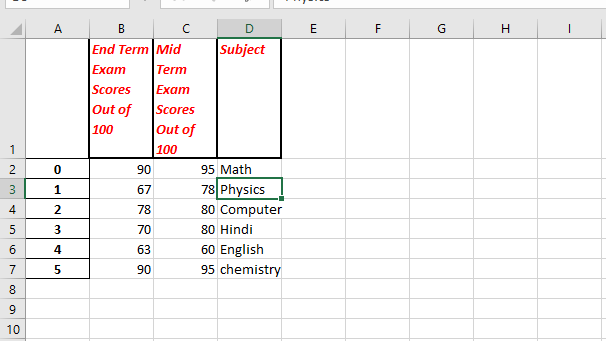# Python | Working with Pandas and XlsxWriter | Set – 2

Prerequisite: : Python working with pandas and xlsxwriter | set-1

Python Pandas is a data analysis library. It can read, filter and re-arrange small and large datasets and output them in a range of formats including Excel.

Pandas writes Excel files using the XlsxWriter modules.

`XlsxWriter` is a Python module for writing files in the XLSX file format. It can be used to write text, numbers, and formulas to multiple worksheets. Also, it supports features such as formatting, images, charts, page setup, auto filters, conditional formatting and many others.

Code #1 : Converting a Pandas dataframe with datetimes to an Excel file with a default datetime and date format using Pandas and XlsxWriter.

 `# import pandas library as pd ` `import` `pandas as pd ` `  `  `# from datetime module import ` `# datetime and date method ` `from` `datetime ``import` `datetime, date ` `  `  `# Create a Pandas dataframe from some datetime data. ` `# datetime(year,month,date,hour,minute,second) ` `# date(year,month,date) ` `dataframe ``=` `pd.DataFrame({ ` `    ``'Date and time'``: [ datetime(``2018``, ``1``, ``11``, ``11``, ``30``, ``55``), ` `                      ``datetime(``2018``, ``2``, ``12``, ``1``,  ``20``, ``33``), ` `                      ``datetime(``2018``, ``3``, ``13``, ``11``, ``10`    `), ` `                      ``datetime(``2018``, ``4``, ``14``, ``16``, ``45``, ``35``), ` `                      ``datetime(``2018``, ``5``, ``15``, ``12``, ``10``, ``15``)], ` `                     `  `   ``'Dates only'``:    [ date(``2018``, ``6``, ``21``), ` `                      ``date(``2018``, ``7``, ``22``), ` `                      ``date(``2018``, ``8``, ``23``), ` `                      ``date(``2018``, ``9``, ``24``), ` `                      ``date(``2018``, ``10``, ``25``) ], }) ` `  `  `# Create a Pandas Excel writer  ` `# object using XlsxWriter as the engine. ` `# Also set the default datetime and date formats. ` `  `  `# mmmm dd yyyy => month date year ` `# month - full name, date - 2 digit, year - 4 digit ` `  `  `# mmm d yyyy hh:mm:ss => month date year hour: minute: second ` `# month - first 3 letters , date - 1 or 2 digit , year - 4 digit. ` `writer_object ``=` `pd.ExcelWriter(``"Example_datetime.xlsx"``, ` `                        ``engine ``=``'xlsxwriter'``, ` `                        ``datetime_format ``=``'mmm d yyyy hh:mm:ss'``, ` `                        ``date_format ``=``'mmmm dd yyyy'``) ` `  `  `# Write a dataframe to the worksheet.  ` `dataframe.to_excel(writer_object, sheet_name ``=``'Sheet1'``) ` `  `  `# Create xlsxwriter worksheet object ` `worksheet_object  ``=` `writer_object.sheets[``'Sheet1'``] ` `  `  `# set width of the B and C column ` `worksheet_object.set_column(``'B:C'``, ``20``) ` `  `  `# Close the Pandas Excel writer  ` `# object and output the Excel file.  ` `writer_object.save() `

Output :Code #2 : Converting a Pandas dataframe to an Excel file with column formats using Pandas and XlsxWriter.

 `# import pandas lib as pd ` `import` `pandas as pd ` `  `  `# Create a Pandas dataframe from some data. ` `dataframe ``=` `pd.DataFrame( ` `    ``{``'Marks (Out of 50)'``: [``30``, ``40``, ``45``, ``15``, ``8``, ``5``, ``35``], ` `     ``'Percentage'``: [.``6``,   .``8``,   .``9``,  .``3``,  .``16``,   .``1``,  .``7` `], }) ` `  `  `# Create a Pandas Excel writer  ` `# object using XlsxWriter as the engine.  ` `writer_object ``=` `pd.ExcelWriter(``"Example_column.xlsx"``, ` `                                ``engine ``=``'xlsxwriter'``) ` `  `  `# Write a dataframe to the worksheet.  ` `dataframe.to_excel(writer_object, sheet_name ``=``'Sheet1'``) ` `  `  `# Create xlsxwriter workbook object . ` `workbook_object ``=` `writer_object.book ` `  `  `# Create xlsxwriter worksheet object ` `worksheet_object ``=` `writer_object.sheets[``'Sheet1'``] ` `  `  `# Create a new Format object to formats cells  ` `# in worksheets using add_format() method . ` `  `  `# number taken upto 2 decimal places ` `# format object is create. ` `format_object1 ``=` `workbook_object.add_format({``'num_format'``: ``'# 0.00'``}) ` `  `  `# Integral percentage format object is create. ` `format_object2 ``=` `workbook_object.add_format({``'num_format'``: ``'0 %'``}) ` `  `  `# Note: It isn't possible to format ` `# any cells that already have a format ` `# such as the index or headers or any ` `# cells that contain dates or datetimes. ` `  `  `# Set the column width and format. ` `worksheet_object.set_column(``'B:B'``, ``20``, format_object1) ` `  `  `# Set the column width and format. ` `worksheet_object.set_column(``'C:C'``, ``15``, format_object2) ` `  `  `# Close the Pandas Excel writer  ` `# object and output the Excel file.  ` `writer_object.save() `

Output :Code #3 : Converting a Pandas dataframe to an Excel file with a user defined header format using Pandas and XlsxWriter.

 `# import pandas lib as pd ` `import` `pandas as pd ` `  `  `  `  `data1 ``=` `[``"Math"``, ``"Physics"``, ``"Computer"``, ` `         ``"Hindi"``, ``"English"``, ``"chemistry"``] ` `data2 ``=` `[``95``, ``78``, ``80``, ``80``, ``60``, ``95``] ` `data3 ``=` `[``90``, ``67``, ``78``, ``70``, ``63``, ``90``] ` `  `  `# Create a Pandas dataframe from some data. ` `dataframe ``=` `pd.DataFrame( ` `    ``{``'Subject'``: data1, ` `     ``'Mid Term Exam Scores Out of 100'` `: data2, ` `     ``'End Term Exam Scores Out of 100'` `: data3}) ` `  `  `# Create a Pandas Excel writer  ` `# object using XlsxWriter as the engine.  ` `writer_object ``=` `pd.ExcelWriter(``"Example_header.xlsx"``, ` `                                ``engine ``=``'xlsxwriter'``) ` `  `  `# Write a dataframe to the worksheet.  ` `# we turn off the default header ` `# and skip one row because we want ` `# to insert a user defined header there. ` `dataframe.to_excel(writer_object, sheet_name ``=``'Sheet1'``,  ` `                          ``startrow ``=` `1``, header ``=` `False``) ` `  `  `# Create xlsxwriter workbook object . ` `workbook_object ``=` `writer_object.book ` `  `  `# Create xlsxwriter worksheet object ` `worksheet_object ``=` `writer_object.sheets[``'Sheet1'``] ` `  `  `# Create a new Format object to formats cells  ` `# in worksheets using add_format() method . ` `  `  `# here we create a format object for header. ` `header_format_object ``=` `workbook_object.add_format({ ` `                                ``'bold'``: ``True``, ` `                                ``'italic'` `: ``True``, ` `                                ``'text_wrap'``: ``True``, ` `                                ``'valign'``: ``'top'``, ` `                                ``'font_color'``: ``'red'``, ` `                                ``'border'``: ``2``}) ` `  `  `# Write the column headers with the defined format. ` `for` `col_number, value ``in` `enumerate``(dataframe.columns.values): ` `    ``worksheet_object.write(``0``, col_number ``+` `1``, value,  ` `                              ``header_format_object) ` `  `  `# Close the Pandas Excel writer  ` `# object and output the Excel file.  ` `writer_object.save() `

Output :My Personal Notes arrow_drop_upCheck out this Author's contributed articles.

If you like GeeksforGeeks and would like to contribute, you can also write an article using contribute.geeksforgeeks.org or mail your article to contribute@geeksforgeeks.org. See your article appearing on the GeeksforGeeks main page and help other Geeks.

Please Improve this article if you find anything incorrect by clicking on the "Improve Article" button below.

Article Tags :

25

Please write to us at contribute@geeksforgeeks.org to report any issue with the above content.